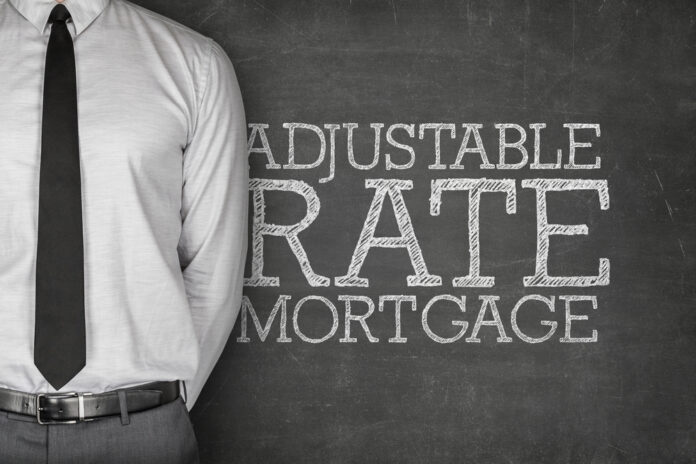# What Exactly Is an Arm Loan?

0
959When it comes to an arm loan (adjustable-rate mortgage), the main thing to know is that the interest rates for the mortgage can vary throughout the life of the loan; they can either be lower than the actual interest rate that the mortgage had or they can be higher than the initial interest.

You do not have to worry about too much volatility, however, because there is a limit as to how much these rates can deviate, and these are things that are federally regulated.

So how can this be of benefit to you? Well, you can use these types of interest strategically if you plan on paying the entire amount of the mortgage quickly before too much deviation can happen on the interest. If you can pay off your balance quickly and the rates start lower than what the fixed rate would have been, then it would be advantageous because that would mean you could have saved yourself thousands of dollars worth of interest accrued!

## Breaking Down Arm Loans

So when you first encounter an arm loan defined on the terms of the mortgage, you will notice that two numbers are used to express the terms of the loan. It would usually be something like 8/25. So what does that mean? The first number is the number of years that a fixed rate for the loan would apply. The second number represents the number of years where the rates can vary. So for our example of 8/25, that would mean that the loan would have a fixed interest rate for eight years and then a variable interest rate over the remaining 25 years.

## How Rates Are Determined

The interest of an arm loan is determined by two things, a variable index rate tied to the rate of financial assets like one-year T-bills. Then there is a margin added on top of it that remains pretty consistent.

If the index rate is at 3% and the margin is at 4%, that means the mortgage has a total rate of 7%. However, if the index rate jumps up to 5%, that would mean that the real interest rate is a whopping 12%.

That is why you have to be careful when deciding to go with interest rates like this. You do not want to have a mortgage like this for an extended period and allow such volatility to potentially hike up your interest rates.

## Things to consider

So now that you know all about arm loans, try to think about whether this is something you could use based on your financial situation. It is not something that is for everyone because they are somewhat risky. If you take a good hard look at the time value of money and you prepare adequately and do not plan to hold these loans for a long time, there is no reason you shouldn’t give them a try!